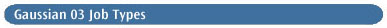#The route section of a Gaussian 03 input file specifies the type of calculation to be performed. There are three key components to this specification:

• The job type

• The method

• The basis set

The following table lists the job types available in Gaussian 03:

• SP     Single point energy.

• Opt     Geometry optimization.

• Freq     Frequency and thermochemical analysis.

• IRC     Reaction path following.

• IRCMax     Find the maximum energy along a specific reaction path.

• Scan     Potential energy surface scan.

• Polar     Polarizabilities and hyperpolarizabilities.

• ADMP and BOMD     Direct dynamics trajectory calculation.

• Force     Compute forces on the nuclei.

• Stable     Test wavefunction stability.

• Volume     Compute molecular volume.

• Density=Checkpoint     Recompute population analysis only.

• Guess=Only     Print initial guess only; recompute population analysis.

• ReArchive     Extract archive entry from checkpoint file only.

In general, only one job type keyword should be specified. The exceptions to this rule are:

• Polar and Opt may be combined with Freq (although SCRF may not be combined with Opt Freq). In the latter case, the geometry optimization is automatically followed by a frequency calculation at the optimized structure.

• Opt may be combined with IRCMax in order to specify options for the optimization portion      of the calculation.

When no job type keyword is specified within the route section, the default calculation type is usually a single point energy calculation (SP). However, a route section of the form: method2/basis2 // method1/basis1 may be used to request an optimization calculation (at method1/basis1) followed by a single point energy calculation (at method2/basis2) at the optimized geometry. For example, the following route section requests a HF/6-31G(d) geometry optimization followed by a single point energy calculation using the QCISD/6-31G(d) model chemistry:

`# QCISD/6-31G(d)//HF/6-31G(d) Test`

In this case, the Opt keyword is optional and is the default. Note that Opt Freq calculations may not use this syntax.

Predicting Molecular Properties The following table provides a mapping between commonly-desired predicted quantities and the Gaussian 03 keywords that will produce them:

• Atomic charges: Pop

• Dipole moment: Pop

• Electron affinities via propagator methods: OVGF

• Electron density: cubegen

• Electronic circular dichroism: TD

• Electrostatic potential: cubegen, Prop

• Electrostatic-potential derived charges: Pop=Chelp, ChelpG or MK

• Frequency-dependent polarizabilities/hyperpolarizabilities: Polar CPHF=RdFreq

• High accuracy energies: CBS-QB3, G2, G3, W1U

• Hyperfine coupling constants (anisotropic): Prop

• Hyperfine spectra tensors (incl. g tensors): Freq=(VCD, VibRot[, Anharmonic])

• Hyperpolarizabilities: Freq, Polar

• Ionization potentials via propagator methods: OVGF

• IR and Raman spectra: Freq

• Pre-resonance Raman spectra: Freq CPHF=RdFreq

• Molecular orbitals: Pop=Regular

• Multipole moments: Pop

• NMR shielding and chemical shifts: NMR

• NMR spin-spin coupling constants: NMR=SpinSpin

• Optical rotations: Polar=OptRot CPHF=RdFreq

• Polarizabilities: Freq, Polar

• Thermochemical analysis: Freq

• UV/Visible spectra: CIS, Zindo, TD

• Vibration-rotation coupling: Freq=VibRot

• Vibrational circular dichroism: Freq=VCD